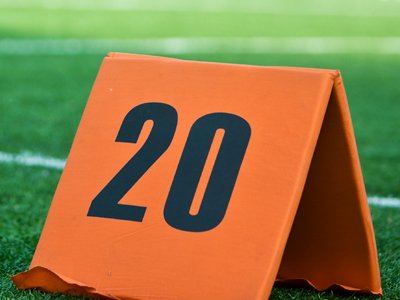Dividing by 120 is the same as multiplying by 20.

# Multiplication and Division 3 (Easy)

Welcome to the third in our Easy section of Eleven Plus maths quizzes on Multiplication and Division. Just like the first two, this quiz will give you more chance to work with decimal numbers and fractions, which can cause some people problems!

• Multiplying by 1 over a number is the same as dividing by that number, so 3 x 15 = 3 ÷ 5 = 35
• To divide by a fraction, invert the fraction and multiply, so , 3 ÷ 25 = 3 x 52 = (3 x 5) ÷ 2 = 7 remainder 1 = 712

Does it still seem a little complicated? Don’t worry. Play the quiz and read all the helpful comments after each question. Keep playing until you can score a perfect 10 out of ten. Good luck!

1.
What is the correct answer to the given calculation?
21 x 17
357
347
337
327
21 × 17 = (20 × 17) + 17 = 357. Decrease 21 by 1 and do the multiplication THEN add 17. Be on the LOOKOUT for products that can be simplified: this will enable you to do the calculation in your head
2.
What is the correct answer to the given calculation?
33 x 22
726
706
686
666
33 x 2 = 66 and 33 x 20 = 660 so 33 x 22 = 66 + 660 = 726. Look for shortcuts like this which allow you to work out the answer in your head
3.
What is the correct answer to the given calculation?
17 x 14
68
414
334
412
Multiplying by 1⁄4 is the same as dividing by 4 ∴ 17 ÷ 4 = 4 remainder 1 = 414. In general, multiplying by 1 over a number is the same as dividing by that number: in this case, 4
4.
What is the correct answer to the given calculation?
3.4 x 120
170
17
1.7
0.17
Remember that multiplying by 1 over a number is the same as dividing that number: in this case, 20:
3.4 ÷ 20 = (3.4 ÷ 2) ÷ 10 = 1.7 ÷ 10 = 0.17
5.
What is the correct answer to the given calculation?
27 ÷ 5
5
515
525
535
27 ÷ 5 = 5 remainder 2. As we are dealing with fifths, the remainder becomes 25
6.
What is the correct answer to the given calculation?
15 x 23
435
425
535
115
1⁄5 × 23 = 23 ÷ 5 = 4 remainder 3 = 435. In general, multiplying by 1 over a number is the same as dividing by that number: in this case, 5
7.
What is the correct answer to the given calculation?
14 ÷ 38
2813
3718
3713
2818
To divide by a fraction, invert (turn upside down) the fraction and multiply. LEARN this technique. So, 14 ÷ 38 = 14 × 83 = (14 × 8) ÷ 3 = 112 ÷ 3 = 37 remainder 1 = 3713
8.
What is the correct answer to the given calculation?
11 ÷ 23
1513
1512
1613
1612
To divide by a fraction, invert (turn upside down) the fraction and multiply. LEARN this technique. So, 11 ÷ 23 = 11 × 32 = (11 × 3) ÷ 2 = 33 ÷ 2 = 16 remainder 1 = 1612
9.
What is the correct answer to the given calculation?
37 ÷ 134
6434
2127
2114
2117
To make this easier, first convert 134 into quarters:
37 ÷ 134 = 37 ÷ 74
Next, invert (turn upside down) the fraction and multiply:
37 ÷ 74 = 37 × 47 = (37 × 4) ÷ 7 = 148 ÷ 7 = 21 remainder 1 = 2117
10.
What is the correct answer to the given calculation?
24 ÷ 12
12
48
36
60
Remember that dividing by 1 over a number is the same as multiplying by that number: in this case, 2: 24 x 2 = 48
Author:  Frank Evans